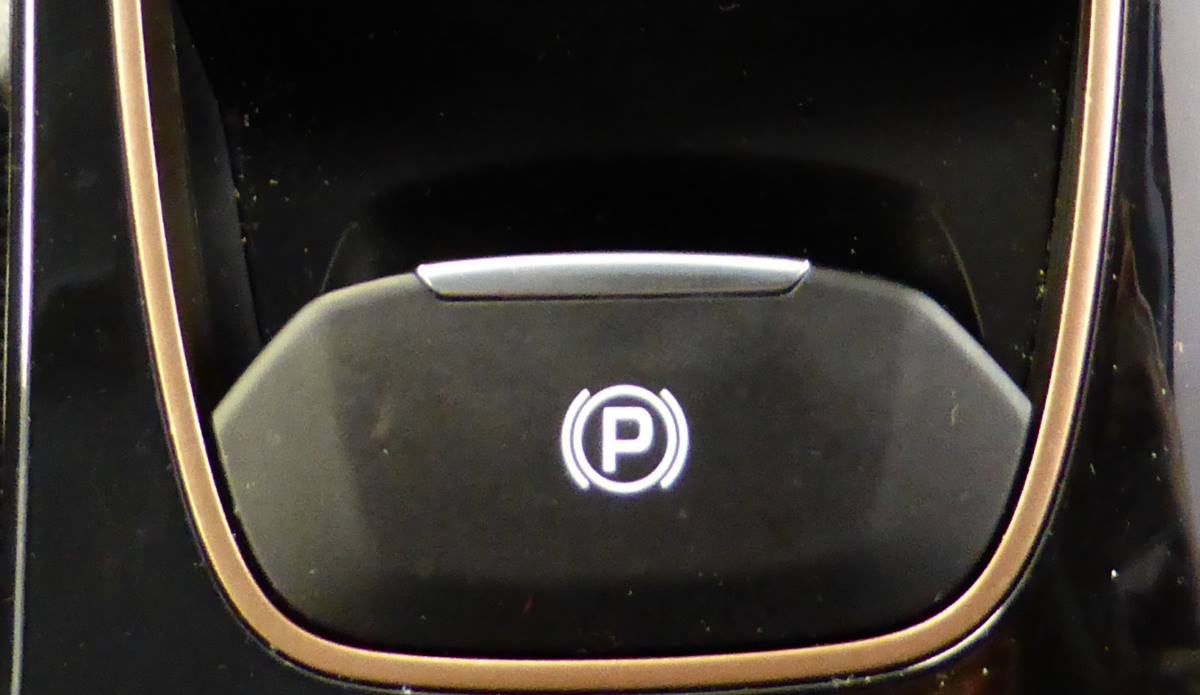# How do you calculate speed vs distance vs time?

Sometimes you’ll need to estimate how long it will take to get somewhere. Maybe you’re planning a journey, maybe you’ve got a maths test. There are quick ways to calculate speed and time.

First, you’ll need to know how to change decimal time into hours, minutes and seconds and vice versa.

To convert standard time into decimal time, let’s take 3 hours 14 minutes 18 seconds. To convert to decimal is straightforward:

18 seconds /60 = 0.3 (i.e. 18 seconds is 0.3 of a minute). Add that to the 14 minutes

14.3 minutes / 60 = 0.238 (i.e. 14 minutes and 18 seconds is 0.238 of an hour)

Add this to the original 3 hours = 3.238 hours.

If you have your time in decimal format already, you do the calculations in reverse. Let’s say we have 2.74 hours. Remove the 2 and you’re left with .74. Multiply this by 60 to convert the decimal time to minutes = 44.4 minutes. Unfortunately, we have 0.4 minutes which doesn’t tell us the exact number of seconds. Take 0.4 and multiply it by 60 to convert to seconds = 24 seconds. Therefore we have 2 hours 44 minutes and 24 seconds.

## How far will a car travel in a certain time, given a certain speed?

If you are calculating in km/h, simply replace mph with km/h.

If a car travels at 60mph, how far away will it be in one hour? The clue is in the question – 60 miles per hour means 60 miles in one hour. However, clarify with the person whether the distance they mean is the distance of the road or the distance as the crow flies. Roads are rarely straight for 60 miles, therefore the person could have driven 60 miles but only be 50 miles away from the initial starting point.

Distance = Speed * Time

For example, a car is travelling at 45 miles per hour for 1 hour 40 minutes.

First, convert 1 hour 40 minutes into decimal time:

40 = 100 minutes. 0.67 hours. Add the 0.67 onto the original hour and you have 1.67 hours.

Therefore distance = 45 * 1.67 = 75 miles.

## How fast is a car travelling if it covers a certain distance in a certain time?

Speed = Distance / Time

For example, a car travels 32 miles in 26 minutes. How fast is it going?

First, convert 26 minutes to hours: 26/60 = 0.43

Therefore 32/0.43 = 73.8 miles per hour.

## How much time will it take for a car to travel a certain distance at a certain speed?

Time = Distance / Speed

For example, a car is travelling at an average of 57mph for 88 miles. How long does it take?

Therefore 88 / 57 = 1.544 hours.

We know we’ve got at least one hour, but we need to convert the 0.544 to minutes. Therefore, multiply 0.544 by 60 = 32.63

Now we have our number of minutes, but it’s still in decimals and we want to know the number of seconds. Take the .63 and multiply by 60 = 37.9

Therefore, the total time taken is 1 hour 32 minutes, 37.9 seconds.

## Other useful conversions

Miles = km / 1.60934

Km = miles * 1.60934

For example, 32 miles in km is 32 * 1.60934 = 51.5

There are 1000 metres in 1 km and 1609.34 metres in 1 mile.Darren has owned several companies in the automotive, advertising and education industries. He has run driving theory educational websites since 2010.

Tagged with: